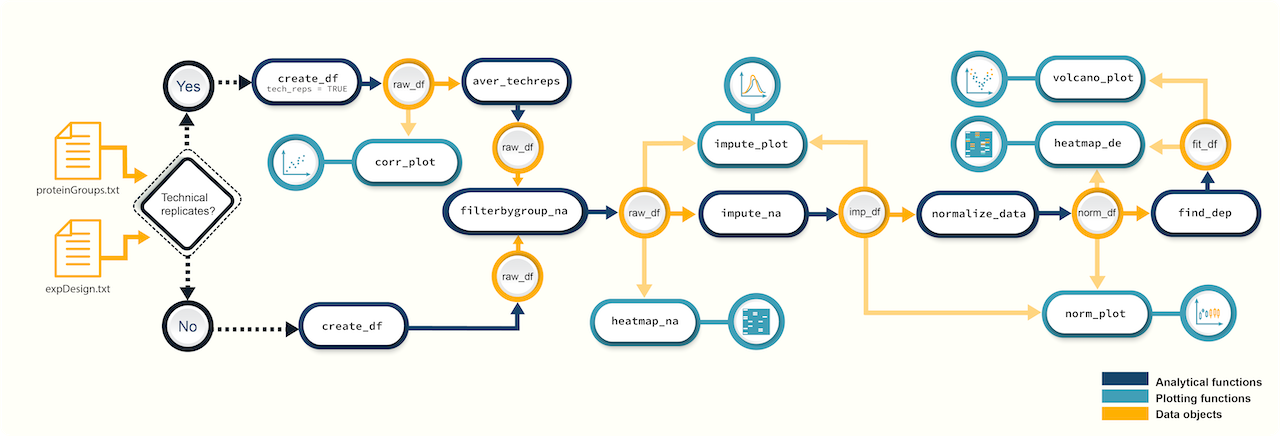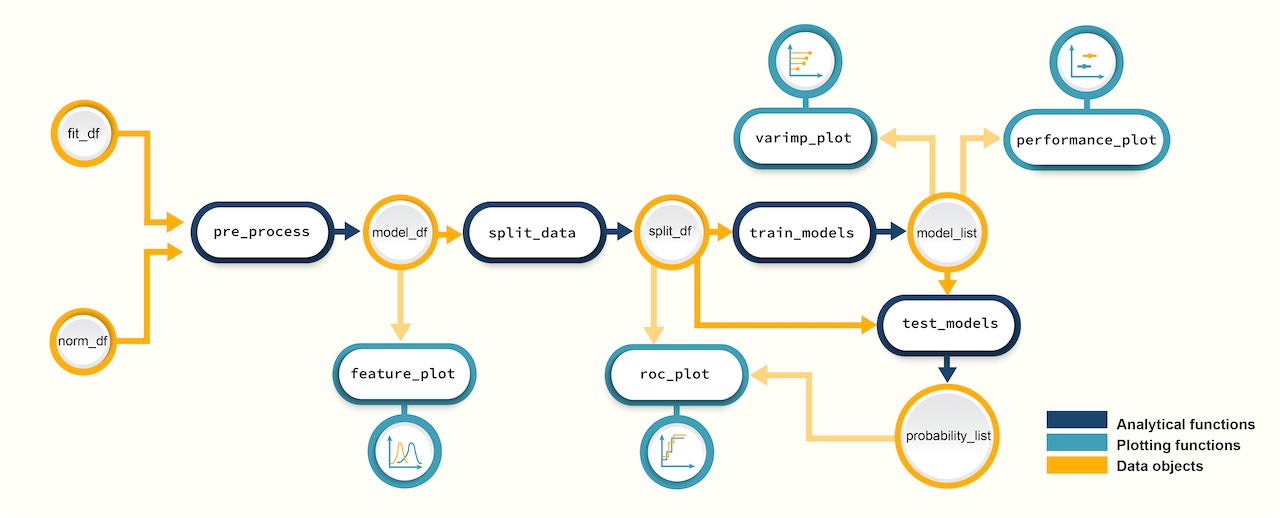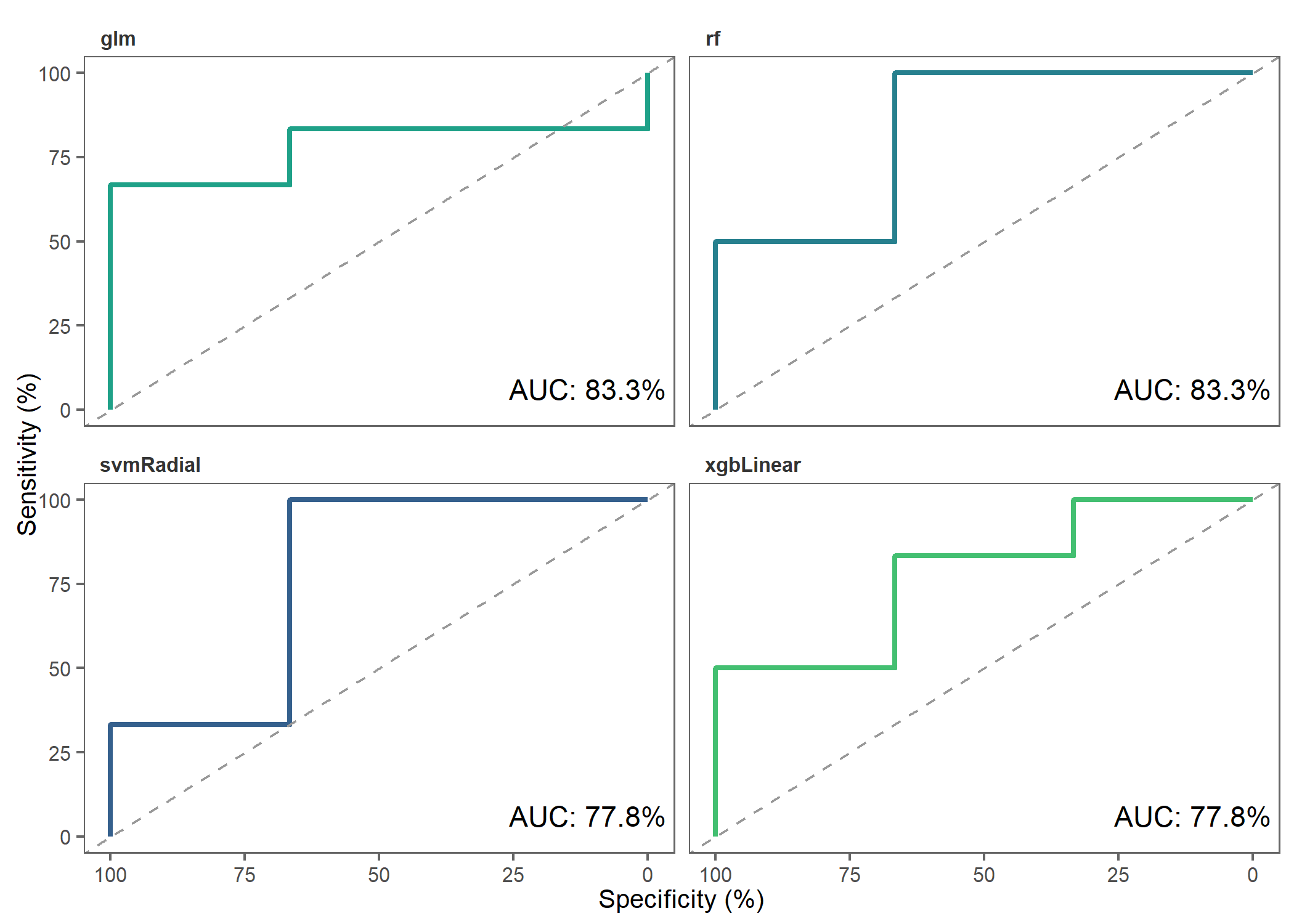### Proteomics Data Analysis and Modeling Tools

• promor is a user-friendly, comprehensive R package that combines proteomics data analysis with machine learning-based modeling.

• promor streamlines differential expression analysis of label-free quantification (LFQ) proteomics data and building predictive models with top protein candidates.

• With promor we provide a range of quality control and visualization tools to analyze label-free proteomics data at the protein level.

• Input files for promor are a proteinGroups.txt file produced by MaxQuant or a standard input file containing a quantitative matrix of protein intensities and an expDesign.txt file containing the experimental design of your proteomics data.

• The standard input file should be a tab-delimited text file. Proteins or protein groups should be indicated by rows and samples by columns. Protein names should be listed in the first column and you may use a column name of your choice for the first column. The remaining sample column names should match the sample names indicated by the mq_label column in the expDesign.txt file.

🚨Check out our R Shiny app: PROMOR App

### Installation

Install the released version from CRAN

install.packages("promor")

Install development version from GitHub

# install devtools, if you haven't already:
install.packages("devtools")

# install promor from github
devtools::install_github("caranathunge/promor")

### Proteomics data analysis with promorFigure 1. A schematic diagram of suggested workflows for proteomics data analysis with promor.

#### Example

Here is a minimal working example showing how to identify differentially expressed proteins between two conditions using promor in five simple steps. We use a previously published data set from Cox et al. (2014) (PRIDE ID: PXD000279).

# Load promor
library(promor)

# Create a raw_df object with the files provided in this github account.
raw <- create_df(
prot_groups = "https://raw.githubusercontent.com/caranathunge/promor_example_data/main/pg1.txt",
exp_design = "https://raw.githubusercontent.com/caranathunge/promor_example_data/main/ed1.txt"
)

# Filter out proteins with high levels of missing data in either condition or group
raw_filtered <- filterbygroup_na(raw)

# Impute missing data and create an imp_df object.
imp_df <- impute_na(raw_filtered)

# Normalize data and create a norm_df object
norm_df <- normalize_data(imp_df)

# Perform differential expression analysis and create a fit_df object
fit_df <- find_dep(norm_df)

Lets take a look at the results using a volcano plot.

volcano_plot(fit_df, text_size = 5)### Modeling with promorFigure 2. A schematic diagram of suggested workflows for building predictive models with promor.

#### Example

The following minimal working example shows you how to use your results from differential expression analysis to build machine learning-based predictive models using promor.

We use a previously published data set from Suvarna et al. (2021) that used differentially expressed proteins between severe and non-severe COVID patients to build models to predict COVID severity.

# First, let's make a model_df object of top differentially expressed proteins.
# We will be using example fit_df and norm_df objects provided with the package.
covid_model_df <- pre_process(
fit_df = covid_fit_df,
norm_df = covid_norm_df
)

# Next, we split the data into training and test data sets
covid_split_df <- split_data(model_df = covid_model_df)

# Let's train our models using the default list of machine learning algorithms
covid_model_list <- train_models(split_df = covid_split_df)

# We can now use our models to predict the test data
covid_prob_list <- test_models(
model_list = covid_model_list,
split_df = covid_split_df
)

Let’s make ROC plots to check how the different models performed.


roc_plot(
probability_list = covid_prob_list,
split_df = covid_split_df
)### Tutorials

You can choose a tutorial from the list below that best fits your experiment and the structure of your proteomics data.

1. This README file can be accessed from RStudio as follows,
vignette("intro_to_promor", package = "promor")
1. If your data do NOT contain technical replicates: promor: No technical replicates

2. If your data contain technical replicates: promor: Technical replicates

3. If you would like to use your proteomics data to build predictive models: promor: Modeling